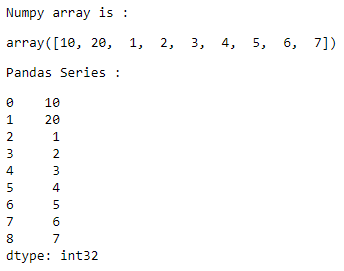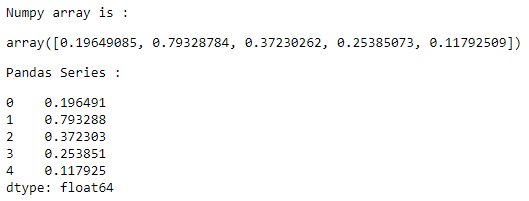Related Articles

# Convert a NumPy array to a Pandas series

• Last Updated : 18 Aug, 2020

Let us see how to convert a NumPy array to a Pandas series. A NumPy array can be converted into a Pandas series by passing it in the `pandas.Series()` function.

Example 1 :

Attention geek! Strengthen your foundations with the Python Programming Foundation Course and learn the basics.

To begin with, your interview preparations Enhance your Data Structures concepts with the Python DS Course. And to begin with your Machine Learning Journey, join the Machine Learning - Basic Level Course

 `# importing the modules``import` `numpy as np``import` `pandas as pd`` ` `# creating an NumPy array``array ``=` `np.array([``10``, ``20``, ``1``, ``2``, ``                   ``3``, ``4``, ``5``, ``6``, ``7``])`` ` `# displaying the NumPy array``print``(``"Numpy array is :"``)``display(array)`` ` `# converting the NumPy array ``# to a Pandas series``series ``=` `pd.Series(array) `` ` `# displaying the Pandas series``print``(``"Pandas Series : "``)``display(series)`

Output :Example 2 :

 `# importing the modules``import` `numpy as np``import` `pandas as pd`` ` `# creating an NumPy array``array ``=` `np.random.rand(``5``) `` ` `# displaying the NumPy array``print``(``"Numpy array is :"``)``display(array)`` ` `# converting the NumPy array ``# to a Pandas series``series ``=` `pd.Series(array) `` ` `# displaying the Pandas series``print``(``"Pandas Series : "``)``display(series)`

Output :My Personal Notes arrow_drop_up